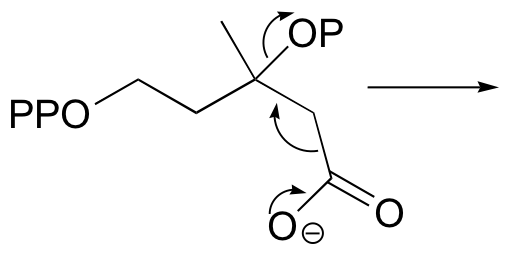Saltar al contenido principal

# 6.7: Problems for Chapter 6

P6.1: In the reactions illustrated below, identify the functional groups which are acting as nucleophile, electrophile, and leaving group.P6.2:  Below is a reaction in the citric acid (Krebs) cycle.a) Draw pictures illustrating the bond-forming and bond-breaking events at each transition state.

b) Identify the nucleophile and the electrophile in the first step.

P6.3: Illustrated below are individual mechanistic steps in some important biochemical reactions that we will be studying later.  For each step, draw the transition state, and also the products or intermediate species that would form according to the electron-movement arrows given. Be sure to include all formal charges.

a)b)c)d)e)f)P6.4: Below is an energy diagram for a hypothetical reaction.  What is the rate limiting step?P6.5: Draw a stereochemically accurate Lewis structure of the peptide with the amino acid sequence CKTV.

$$\newcommand{\vecs}{\overset { \rightharpoonup} {\mathbf{#1}} }$$ $$\newcommand{\vecd}{\overset{-\!-\!\rightharpoonup}{\vphantom{a}\smash {#1}}}$$$$\newcommand{\id}{\mathrm{id}}$$ $$\newcommand{\Span}{\mathrm{span}}$$ $$\newcommand{\kernel}{\mathrm{null}\,}$$ $$\newcommand{\range}{\mathrm{range}\,}$$ $$\newcommand{\RealPart}{\mathrm{Re}}$$ $$\newcommand{\ImaginaryPart}{\mathrm{Im}}$$ $$\newcommand{\Argument}{\mathrm{Arg}}$$ $$\newcommand{\norm}{\| #1 \|}$$ $$\newcommand{\inner}{\langle #1, #2 \rangle}$$ $$\newcommand{\Span}{\mathrm{span}}$$ $$\newcommand{\id}{\mathrm{id}}$$ $$\newcommand{\Span}{\mathrm{span}}$$ $$\newcommand{\kernel}{\mathrm{null}\,}$$ $$\newcommand{\range}{\mathrm{range}\,}$$ $$\newcommand{\RealPart}{\mathrm{Re}}$$ $$\newcommand{\ImaginaryPart}{\mathrm{Im}}$$ $$\newcommand{\Argument}{\mathrm{Arg}}$$ $$\newcommand{\norm}{\| #1 \|}$$ $$\newcommand{\inner}{\langle #1, #2 \rangle}$$ $$\newcommand{\Span}{\mathrm{span}}$$